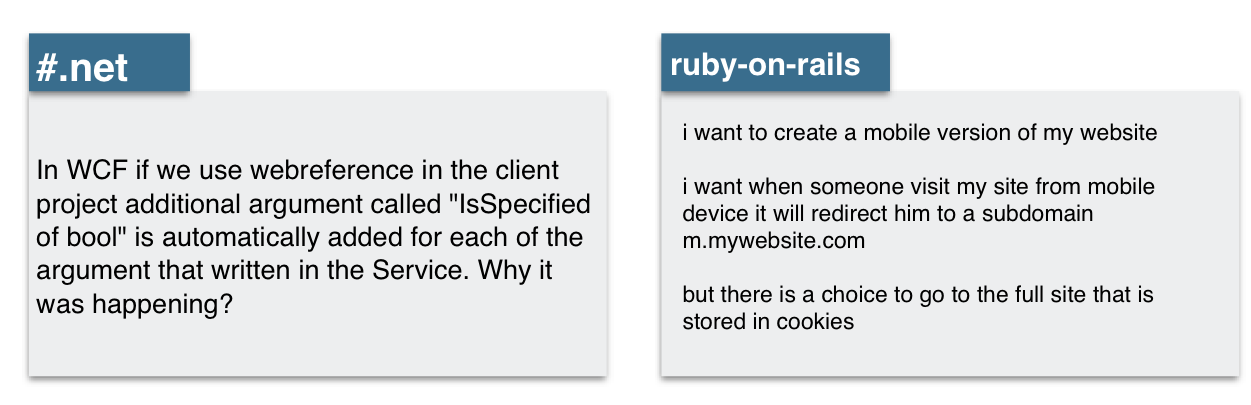Francisca Dias

## Introduction¶

In :
from IPython.display import Image
Image("stack_overflow.png")

Out:Above are two questions that were asked on Stack Overflow. The first has a tag #.net and the second one is related to ruby-on-rails.

In this report I will build a model that can predict the tag on new questions on Stack Overflow.

In this dataset there are 20 tags.

Each tag corresponds to a programming language or a topic related to technology:

c#, python, css, javascript, androind, iphone, ios, etc.

This dataset can be found here.

In :
import pandas as pd

In :
data = pd.read_csv("stack-overflow-data.csv")

In :
data.head()

Out:
post tags
0 what is causing this behavior in our c# datet... c#
1 have dynamic html load as if it was in an ifra... asp.net
2 how to convert a float value in to min:sec i ... objective-c
3 .net framework 4 redistributable just wonderi... .net
4 trying to calculate and print the mean and its... python

How many instances our data has?

In :
len(data)

Out:
40000

Split data into train and test

In :
train_size = int(len(data) * .8)
print(train_size)

32000

In :
print ("Train size: %d" % train_size)
print ("Test size: %d" % (len(data) - train_size))

Train size: 32000
Test size: 8000

In :
train_comments = data['post'][:train_size]
train_labels = data['tags'][:train_size]

test_comments = data['post'][train_size:]
test_labels = data['tags'][train_size:]


## Processing features in Keras¶

We have to process our features in a format that Keras can read

In :
from keras.preprocessing import text

max_words = 1000
t = text.Tokenizer(num_words=max_words, char_level=False)

Using TensorFlow backend.
/Users/FranciscaDias/anaconda/lib/python3.6/importlib/_bootstrap.py:219: RuntimeWarning: compiletime version 3.5 of module 'tensorflow.python.framework.fast_tensor_util' does not match runtime version 3.6
return f(*args, **kwds)


Fit on train data

In :
t.fit_on_texts(train_comments)


There are 129402 distinct words on X_train

In :
print("There are %d" % len(t.word_counts), "distinct words on X_train")
print("In: %d" %t.document_count, "documents or instances")

There are 129402 distinct words on X_train
In: 32000 documents or instances


Documents have to be encoded using the Tokenizer by calling texts to matrix()

In :
X_train = t.texts_to_matrix(train_comments)
X_test = t.texts_to_matrix(test_labels)


## Processing labels in Keras¶

Use LabelBinarizer class to convert label strings to numbered

In :
from sklearn.preprocessing import LabelEncoder

encoder = LabelEncoder()
encoder.fit(train_labels)
y_train = encoder.transform(train_labels)
y_test = encoder.transform(test_labels)

In :
print('X_train shape:', X_train.shape)
print('X_test shape:', X_test.shape)
print('y_train shape:', y_train.shape)
print('y_test shape:', y_test.shape)

X_train shape: (32000, 1000)
X_test shape: (8000, 1000)
y_train shape: (32000,)
y_test shape: (8000,)


Now let us convert the labels to a one-hot representation

In :
# Converts the labels to a one-hot representation
import numpy as np
from keras import utils

num_classes = np.max(y_train) + 1

y_train = utils.to_categorical(y_train, num_classes)
y_test = utils.to_categorical(y_test, num_classes)

In :
print('x_train shape:', X_train.shape)
print('x_test shape:', X_test.shape)
print('y_train shape:', y_train.shape)
print('y_test shape:', y_test.shape)

x_train shape: (32000, 1000)
x_test shape: (8000, 1000)
y_train shape: (32000, 20)
y_test shape: (8000, 20)


## Build the model¶

In :
from keras.models import Sequential

model = Sequential()

In :
from keras.layers import Dense, Activation, Dropout

max_words = 1000

model.add(Dense(512, input_shape=(max_words,)))
model.add(Activation('relu'))
model.add(Dropout(0.5))
model.add(Dense(num_classes))
model.add(Activation('softmax'))

model.compile(loss='categorical_crossentropy',
optimizer='adam',
metrics=['accuracy'])


Now we’re ready to add our input layer, that is called Dense layer, which means each neuron in this layer will be fully connected to all neurons in the next layer.

We pass the Dense layer two parameters:

• 1- the dimensionality of the layer’s output (number of neurons): It’s common to use a power of 2 as the number of dimensions, so we’ll start with 512.

• 2- the shape of our input data: max_words (equals 1000) arrays for each comment

The number of rows in our input data will be the number of posts we’re feeding the model at each training step (called batch size),

and the number of columns will be the size of our vocabulary.

With that, we’re ready to define the Dense input layer.

The activation function tells our model how to calculate the output of a layer (you can read more about ReLU here).

In :
history = model.fit(X_train, y_train,
batch_size=32,
epochs=2,
verbose=1,
validation_split=0.1)

Train on 28800 samples, validate on 3200 samples
Epoch 1/2
28800/28800 [==============================] - 11s 384us/step - loss: 0.9788 - acc: 0.7188 - val_loss: 0.6005 - val_acc: 0.8153
Epoch 2/2
28800/28800 [==============================] - 9s 327us/step - loss: 0.5502 - acc: 0.8248 - val_loss: 0.5892 - val_acc: 0.8122


Evaluate the model

In :
score = model.evaluate(X_test, y_test,
batch_size=32, verbose=1)
print('Test score:', score)
print('Test accuracy:', score)

8000/8000 [==============================] - 1s 70us/step
Test score: 0.436044389367
Test accuracy: 0.90175


## Predicitions on real examples¶

Lets make predicitions on real examples

Remember that test_comments contained all the comments text:

In :
test_comments.head()

Out:
32000    jquery( tr_selector ) is removing the style va...
32001    web reference in wcf  in wcf if we use webrefe...
32002    fragment transaction custom animation - androi...
32003    why can i not use the namespace directive in c...
32004    generating password reset link in c# .net  i m...
Name: post, dtype: object

Let us see how well the model predicts the first five comments

Let´s make predicitions on new data, that is, on test data, for the first 5 comments:

In :
for i in range(5):
prediction = model.predict(np.array([X_test[i]]))
predicted_label = encoder.classes_[np.argmax(prediction)]
print(test_comments.iloc[i][:50], "...")
print('Actual label:' + test_labels.iloc[i])
print("Predicted label: " + predicted_label + "\n")

jquery( tr_selector ) is removing the style values ...
Actual label:jquery
Predicted label: jquery

web reference in wcf  in wcf if we use webreferenc ...
Actual label:.net
Predicted label: .net

fragment transaction custom animation - android  i ...
Actual label:android
Predicted label: android

why can i not use the namespace directive in c++ s ...
Actual label:c++
Predicted label: c#

generating password reset link in c# .net  i must  ...
Actual label:.net
Predicted label: .net



It looks like in 5 questions we only missed one. The fourth question is related to c++ but the model predicted c#.